Main Content

# Tuning of a Two-Loop Autopilot

This example shows how to use Simulink Control Design to tune a two-loop autopilot controlling the pitch rate and vertical acceleration of an airframe.

### Model of Airframe Autopilot

The airframe dynamics and the autopilot are modeled in Simulink.

open_system('rct_airframe1')The autopilot consists of two cascaded loops. The inner loop controls the pitch rate q, and the outer loop controls the vertical acceleration az in response to the pilot stick command azref. In this architecture, the tunable elements include the PI controller gains ("az Control" block) and the pitch-rate gain ("q Gain" block). The autopilot must be tuned to respond to a step command azref in about 1 second with minimal overshoot. In this example, we tune the autopilot gains for one flight condition corresponding to zero incidence and a speed of 984 m/s.

To analyze the airframe dynamics, trim the airframe forand. The trim condition corresponds to zero normal acceleration and pitching moment (andsteady). Use findop to compute the corresponding closed-loop operating condition. Note that we added a "delta trim" input port so that findop can adjust the fin deflection to produce the desired equilibrium of forces and moments.

opspec = operspec('rct_airframe1'); % Specify trim condition % Xe,Ze: known, not steady opspec.States(1).Known = [1;1]; opspec.States(1).SteadyState = [0;0]; % u,w: known, w steady opspec.States(3).Known = [1 1]; opspec.States(3).SteadyState = [0 1]; % theta: known, not steady opspec.States(2).Known = 1; opspec.States(2).SteadyState = 0; % q: unknown, steady opspec.States(4).Known = 0; opspec.States(4).SteadyState = 1; % integrator states unknown, not steady opspec.States(5).SteadyState = 0; opspec.States(6).SteadyState = 0; op = findop('rct_airframe1',opspec); 
 Operating point search report: --------------------------------- Operating point search report for the Model rct_airframe1. (Time-Varying Components Evaluated at time t=0) Operating point specifications were successfully met. States: ---------- (1.) rct_airframe1/Airframe Model/Aerodynamics & Equations of Motion/ Equations of Motion (Body Axes)/Position x: 0 dx: 984 x: -3.05e+03 dx: 0 (2.) rct_airframe1/Airframe Model/Aerodynamics & Equations of Motion/ Equations of Motion (Body Axes)/Theta x: 0 dx: -0.00972 (3.) rct_airframe1/Airframe Model/Aerodynamics & Equations of Motion/ Equations of Motion (Body Axes)/U,w x: 984 dx: 22.7 x: 0 dx: -1.44e-11 (0) (4.) rct_airframe1/Airframe Model/Aerodynamics & Equations of Motion/ Equations of Motion (Body Axes)/q x: -0.00972 dx: 1.15e-16 (0) (5.) rct_airframe1/Integrator x: 0.000708 dx: -0.00972 (6.) rct_airframe1/az Control/Integrator/Continuous/Integrator x: 0 dx: 0.000242 Inputs: ---------- (1.) rct_airframe1/delta trim u: 0.000708 [-Inf Inf] Outputs: None ---------- 

Linearize the "Airframe Model" block for the computed trim condition op and plot the gains from the fin deflection delta to az and q:

G = linearize('rct_airframe1','rct_airframe1/Airframe Model',op); G.InputName = 'delta'; G.OutputName = {'az','q'}; bodemag(G), gridNote that the airframe model has an unstable pole:

pole(G) 
ans = -0.0320 -0.0255 0.1253 -29.4685 

### Frequency-Domain Tuning with LOOPTUNE

You can use the looptune function to automatically tune multi-loop control systems subject to basic requirements such as integral action, adequate stability margins, and desired bandwidth. To apply looptune to the autopilot model, create an instance of the slTuner interface and designate the Simulink blocks "az Control" and "q Gain" as tunable. Also specify the trim condition op to correctly linearize the airframe dynamics.

ST0 = slTuner('rct_airframe1',{'az Control','q Gain'},op); 

Mark the reference, control, and measurement signals as points of interest for analysis and tuning.

addPoint(ST0,{'az ref','delta fin','az','q'}); 

Finally, tune the control system parameters to meet the 1 second response time requirement. In the frequency domain, this roughly corresponds to a gain crossover frequency wc = 5 rad/s for the open-loop response at the plant input "delta fin".

wc = 5; Controls = 'delta fin'; Measurements = {'az','q'}; [ST,gam,Info] = looptune(ST0,Controls,Measurements,wc); 
Final: Peak gain = 1.01, Iterations = 72 

The requirements are normalized so a final value near 1 means that all requirements are met. Confirm this by graphically validating the design.

figure('Position',[100,100,560,714]) loopview(ST,Info)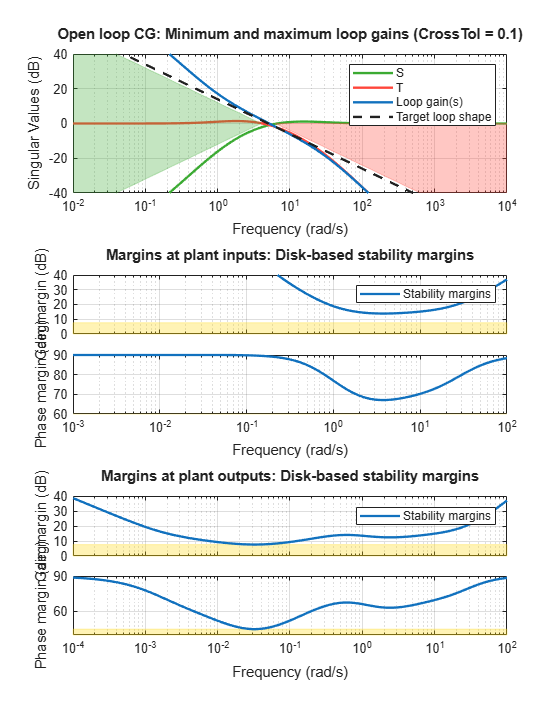The first plot confirms that the open-loop response has integral action and the desired gain crossover frequency while the second plot shows that the MIMO stability margins are satisfactory (the blue curve should remain below the yellow bound). Next check the response from the step command azref to the vertical acceleration az:

T = getIOTransfer(ST,'az ref','az'); figure step(T,5)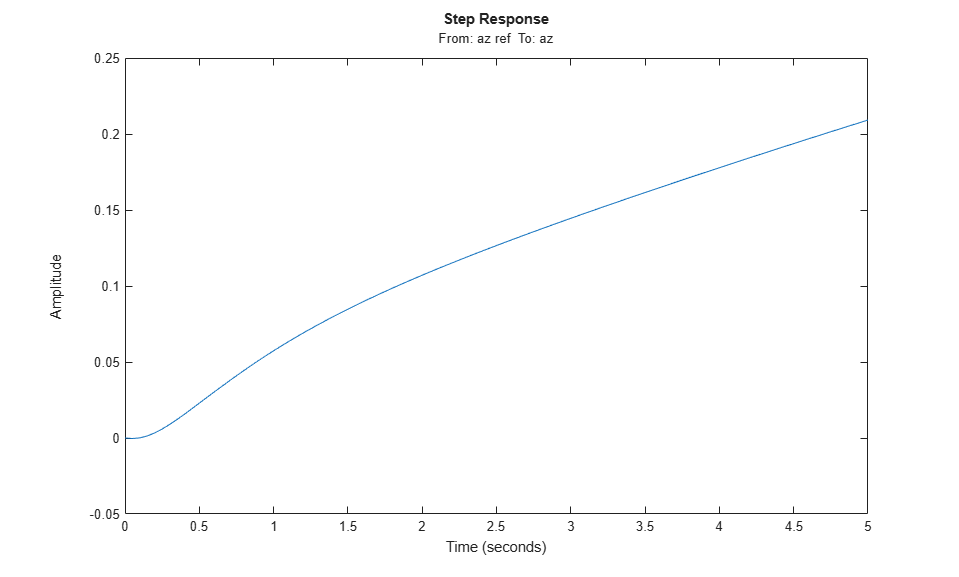The acceleration az does not track azref despite the presence of an integrator in the loop. This is because the feedback loop acts on the two variables az and q and we have not specified which one should track azref.

### Adding a Tracking Requirement

To remedy this issue, add an explicit requirement that az should follow the step command azref with a 1 second response time. Also relax the gain crossover requirement to the interval [3,12] to let the tuner find the appropriate gain crossover frequency.

TrackReq = TuningGoal.Tracking('az ref','az',1); ST = looptune(ST0,Controls,Measurements,[3,12],TrackReq); 
Final: Peak gain = 1.23, Iterations = 54 

The step response from azref to az is now satisfactory:

Tr1 = getIOTransfer(ST,'az ref','az'); step(Tr1,5) grid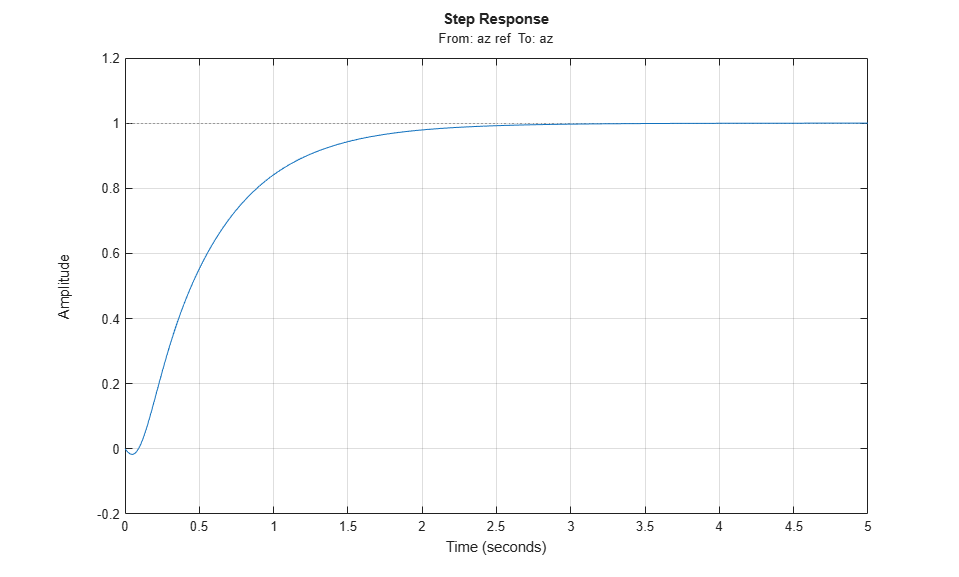Also check the disturbance rejection characteristics by looking at the responses from a disturbance entering at the plant input

Td1 = getIOTransfer(ST,'delta fin','az'); bodemag(Td1) grid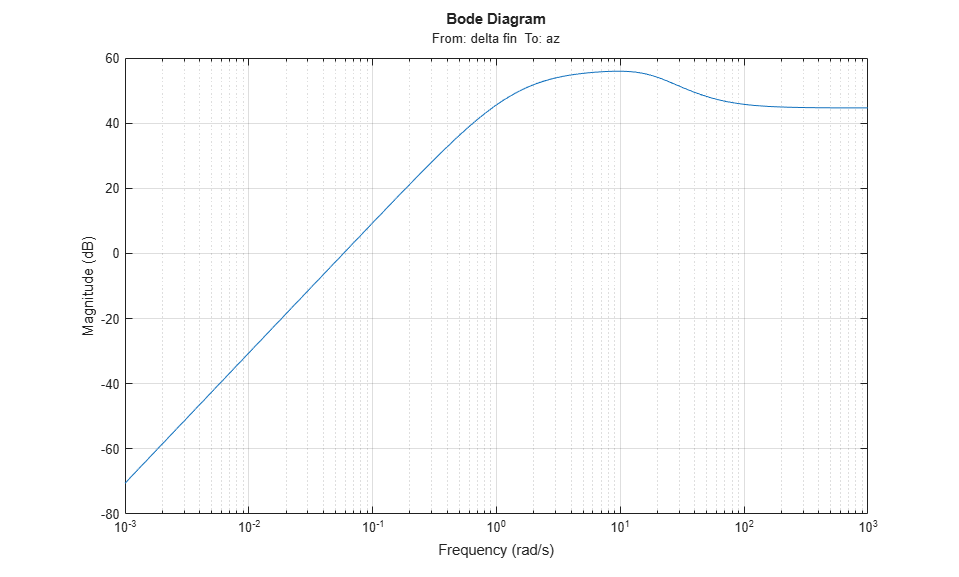step(Td1,5) grid title('Disturbance rejection')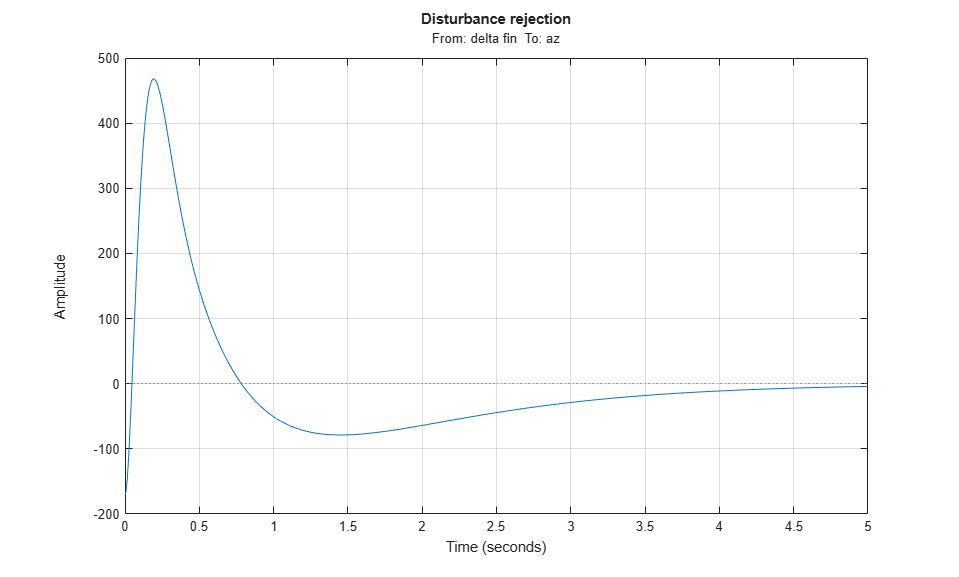Use showBlockValue to see the tuned values of the PI controller and inner-loop gain

showBlockValue(ST) 
AnalysisPoints_ = D = u1 u2 u3 u4 y1 1 0 0 0 y2 0 1 0 0 y3 0 0 1 0 y4 0 0 0 1 Name: AnalysisPoints_ Static gain. ----------------------------------- az_Control = 1 Kp + Ki * --- s with Kp = 0.00166, Ki = 0.0017 Name: az_Control Continuous-time PI controller in parallel form. ----------------------------------- q_Gain = D = u1 y1 1.985 Name: q_Gain Static gain. 

If this design is satisfactory, use writeBlockValue to apply the tuned values to the Simulink model and simulate the tuned controller in Simulink.

writeBlockValue(ST) 

### MIMO Design with SYSTUNE

Cascaded loops are commonly used for autopilots. Yet one may wonder how a single MIMO controller that uses both az and q to generate the actuator command delta fin would compare with the two-loop architecture. Trying new control architectures is easy with systune or looptune. For variety, we now use systune to tune the following MIMO architecture.

open_system('rct_airframe2')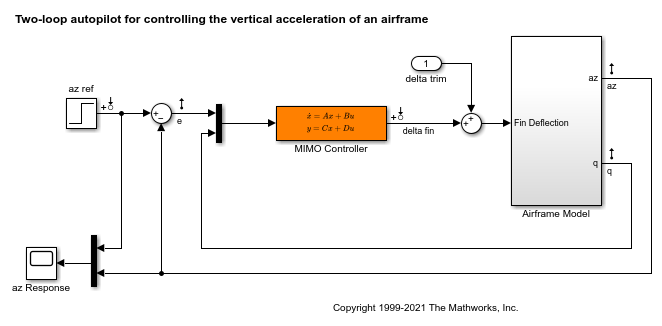As before, compute the trim condition forand.

opspec = operspec('rct_airframe2'); % Specify trim condition % Xe,Ze: known, not steady opspec.States(1).Known = [1;1]; opspec.States(1).SteadyState = [0;0]; % u,w: known, w steady opspec.States(3).Known = [1 1]; opspec.States(3).SteadyState = [0 1]; % theta: known, not steady opspec.States(2).Known = 1; opspec.States(2).SteadyState = 0; % q: unknown, steady opspec.States(4).Known = 0; opspec.States(4).SteadyState = 1; % controller states unknown, not steady opspec.States(5).SteadyState = [0;0]; op = findop('rct_airframe2',opspec); 
 Operating point search report: --------------------------------- Operating point search report for the Model rct_airframe2. (Time-Varying Components Evaluated at time t=0) Operating point specifications were successfully met. States: ---------- (1.) rct_airframe2/Airframe Model/Aerodynamics & Equations of Motion/ Equations of Motion (Body Axes)/Position x: 0 dx: 984 x: -3.05e+03 dx: 0 (2.) rct_airframe2/Airframe Model/Aerodynamics & Equations of Motion/ Equations of Motion (Body Axes)/Theta x: 0 dx: -0.00972 (3.) rct_airframe2/Airframe Model/Aerodynamics & Equations of Motion/ Equations of Motion (Body Axes)/U,w x: 984 dx: 22.7 x: 0 dx: 2.46e-11 (0) (4.) rct_airframe2/Airframe Model/Aerodynamics & Equations of Motion/ Equations of Motion (Body Axes)/q x: -0.00972 dx: -1.72e-16 (0) (5.) rct_airframe2/MIMO Controller x: 0.000654 dx: -0.009 x: 3.76e-20 dx: 0.0303 Inputs: ---------- (1.) rct_airframe2/delta trim u: 0.000436 [-Inf Inf] Outputs: None ---------- 

As with looptune, use the slTuner interface to configure the Simulink model for tuning. Note that the signals of interest are already marked as Linear Analysis points in the Simulink model.

ST0 = slTuner('rct_airframe2','MIMO Controller',op); 

Try a second-order MIMO controller with zero feedthrough from e to delta fin. To do this, create the desired controller parameterization and associate it with the "MIMO Controller" block using setBlockParam:

C0 = tunableSS('C',2,1,2); % Second-order controller C0.D.Value(1) = 0; % Fix D(1) to zero C0.D.Free(1) = false; setBlockParam(ST0,'MIMO Controller',C0) 

Next create the tuning requirements. Here we use the following four requirements:

1. Tracking: az should respond in about 1 second to the azref command

2. Bandwidth and roll-off: The loop gain at delta fin should roll off after 25 rad/s with a -20 dB/decade slope

3. Stability margins: The margins at delta fin should exceed 7 dB and 45 degrees

4. Disturbance rejection: The attenuation factor for input disturbances should be 40 dB at 1 rad/s increasing to 100 dB at 0.001 rad/s.

% Tracking Req1 = TuningGoal.Tracking('az ref','az',1); % Bandwidth and roll-off Req2 = TuningGoal.MaxLoopGain('delta fin',tf(25,[1 0])); % Margins Req3 = TuningGoal.Margins('delta fin',7,45); % Disturbance rejection % Use an FRD model to sketch the desired attenuation profile with a few points Freqs = [0 0.001 1]; MinAtt = [100 100 40]; % in dB Req4 = TuningGoal.Rejection('delta fin',frd(db2mag(MinAtt),Freqs)); Req4.Focus = [0 1]; 

You can now use systune to tune the controller parameters subject to these requirements.

AllReqs = [Req1,Req2,Req3 Req4]; Opt = systuneOptions('RandomStart',3); rng(0) [ST,fSoft] = systune(ST0,AllReqs,Opt); 
Final: Soft = 1.42, Hard = -Inf, Iterations = 47 Final: Soft = 1.42, Hard = -Inf, Iterations = 62 Final: Soft = 1.14, Hard = -Inf, Iterations = 84 Final: Soft = 1.14, Hard = -Inf, Iterations = 102 

The best design has an overall objective value close to 1, indicating that all four requirements are nearly met. Use viewGoal to inspect each requirement for the best design.

figure('Position',[100,100,987,474]) viewGoal(AllReqs,ST)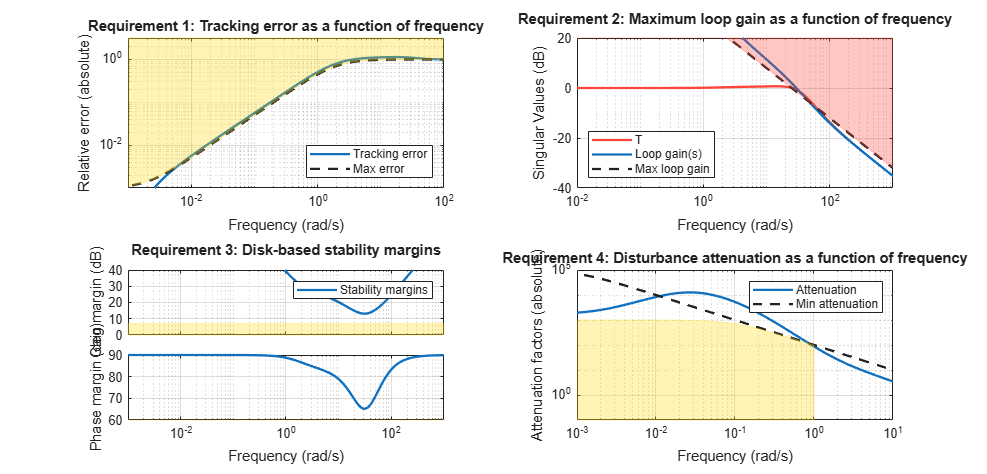Compute the closed-loop responses and compare with the two-loop design.

T = getIOTransfer(ST,{'az ref','delta fin'},'az'); figure step(Tr1,'b',T(1),'r',5) title('Tracking') legend('Cascade','2 dof')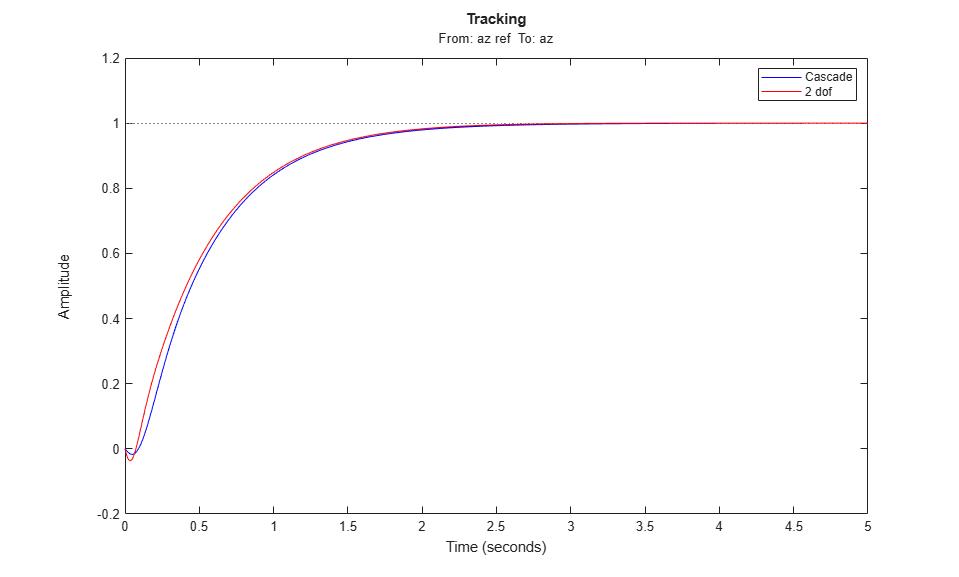step(Td1,'b',T(2),'r',5) title('Disturbance rejection') legend('Cascade','2 dof')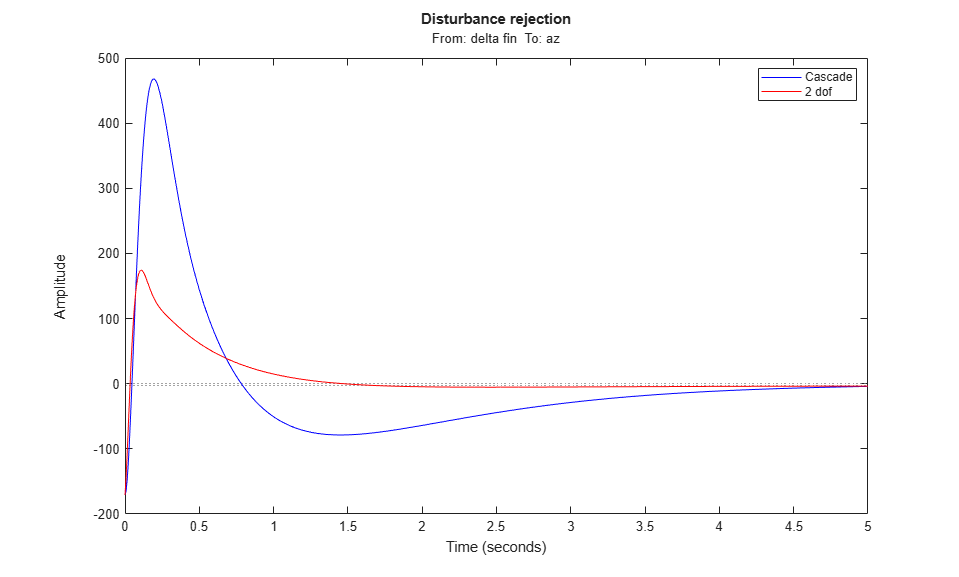The tracking performance is similar but the second design has better disturbance rejection properties.

## SupportGet trial now